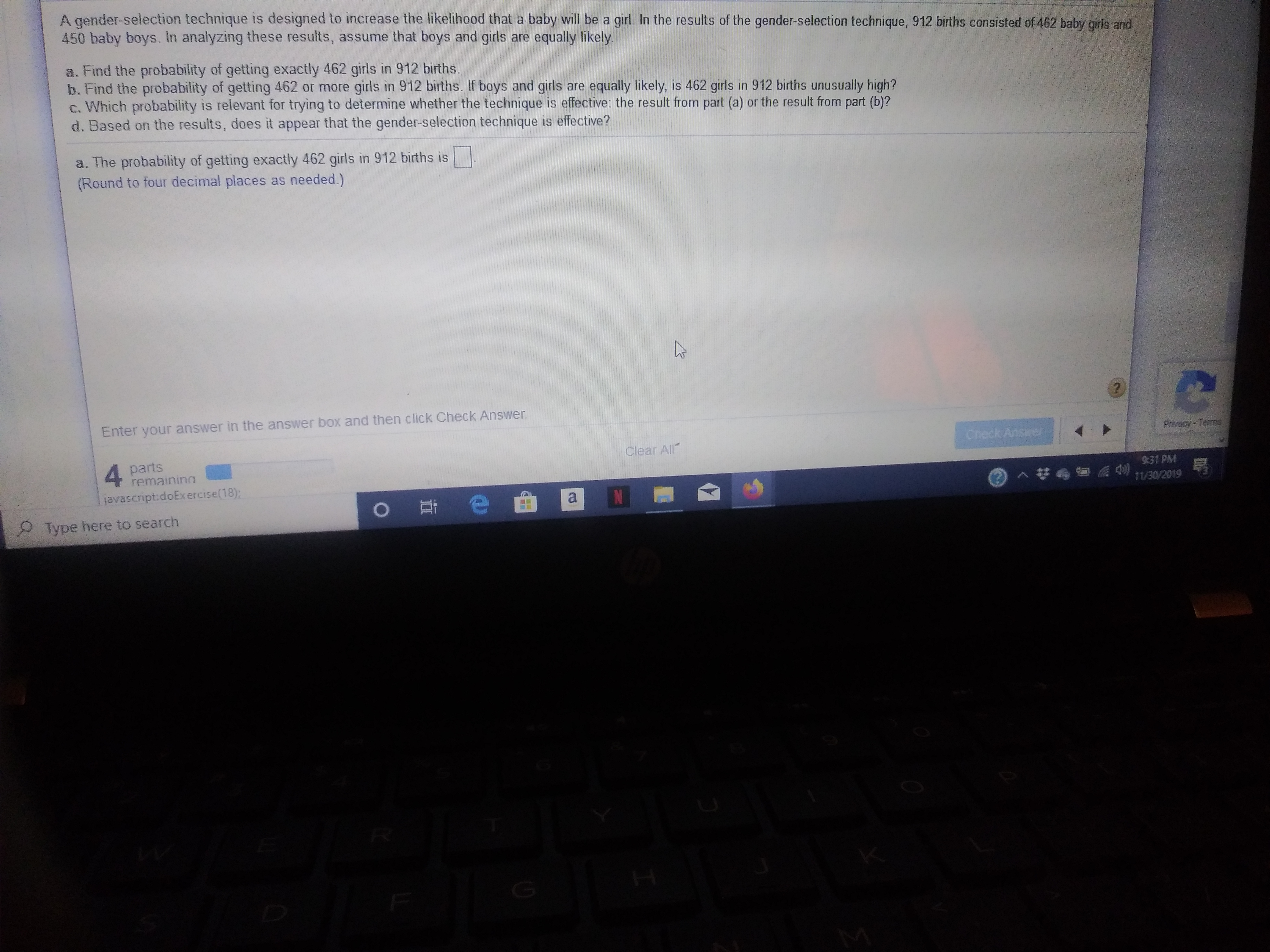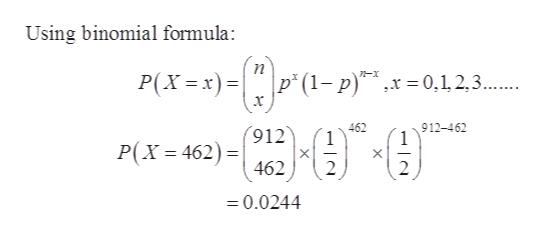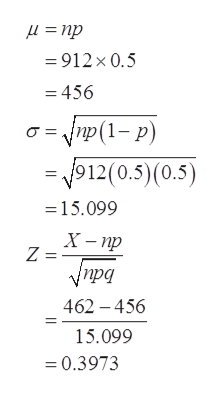# A qender-selection technique is designed to increase the likelihood that a baby will be a girl. In the results of the gender-selection technique, 912 births consisted of 462 baby girls and450 baby boys. In analyzing these results, assume that boys and girls are equally likelya. Find the probability of getting exactly 462 girls in 912 births.b. Find the probability of getting 462 or more girls in 912 births. If boys and girls are equally likely, is 462 girls in 912 births unusually high?c. Which probability is relevant for trying to determine whether the technique is effective: the result from part (a) or the result from part (b)?d. Based on the results, does it appear that the gender-selection technique is effective?a. The probability of getting exactly 462 girls in 912 births is(Round to four decimal places as needed.)Enter your answer in the answer box and then click Check Answer.Privacy TermsCheck Ansirer4 partsremainingClear All931 PMjavascript:doExercise(18);NO e11/30/2019Type here to search

Question
12 viewshelp_outlineImage TranscriptioncloseA qender-selection technique is designed to increase the likelihood that a baby will be a girl. In the results of the gender-selection technique, 912 births consisted of 462 baby girls and 450 baby boys. In analyzing these results, assume that boys and girls are equally likely a. Find the probability of getting exactly 462 girls in 912 births. b. Find the probability of getting 462 or more girls in 912 births. If boys and girls are equally likely, is 462 girls in 912 births unusually high? c. Which probability is relevant for trying to determine whether the technique is effective: the result from part (a) or the result from part (b)? d. Based on the results, does it appear that the gender-selection technique is effective? a. The probability of getting exactly 462 girls in 912 births is (Round to four decimal places as needed.) Enter your answer in the answer box and then click Check Answer. Privacy Terms Check Ansirer 4 parts remaining Clear All 931 PM javascript:doExercise(18); N O e 11/30/2019 Type here to search fullscreen
check_circle

Step 1

From the given information,

Total number of births = 912

Total number of baby girl birth = 462

Total number of baby boy birth = 450

Since, births are independent of gender that is baby will be a girl or boy are equally likely to happen.

Thus,

P(GIRL) = P(BOY)

= 1/2

Let x be a random variable that represents the born baby will be a girl.

So, in general x

From the given information,

Total number of births = 912

Total number of baby girl birth = 462

Total number of baby boy birth = 450

Since, births are independent of gender that is baby will be a girl or boy are equally likely to happen.

Thus,

P(GIRL) = P(BOY)

= 1/2

Let x be a random variable that represents the born baby will be a girl.

So, in general x follows binomial distribution.

Thus, required probability is –

follows binomial distribution.

Step 2

Thus, required probability is –help_outlineImage TranscriptioncloseUsing binomial formula: P(X x= p (1-p)x0,1, 2,3.... x 912-462 1 462 1 X 2 912 P(X 462) X 462 2 =0.0244 fullscreen
Step 3

Since, np≥10 or at least np is 5 (np>5) therefore the binomial distribution can be treat...help_outlineImage Transcriptioncloseи - пр 912 x 0.5 = 456 amp(1-P) 912(0.5)(0.5) =15.099 X-пр Z = 462 -456 15.099 0.3973 fullscreen

### Want to see the full answer?

See Solution

#### Want to see this answer and more?

Solutions are written by subject experts who are available 24/7. Questions are typically answered within 1 hour.*

See Solution
*Response times may vary by subject and question.
Tagged in

### Statistics